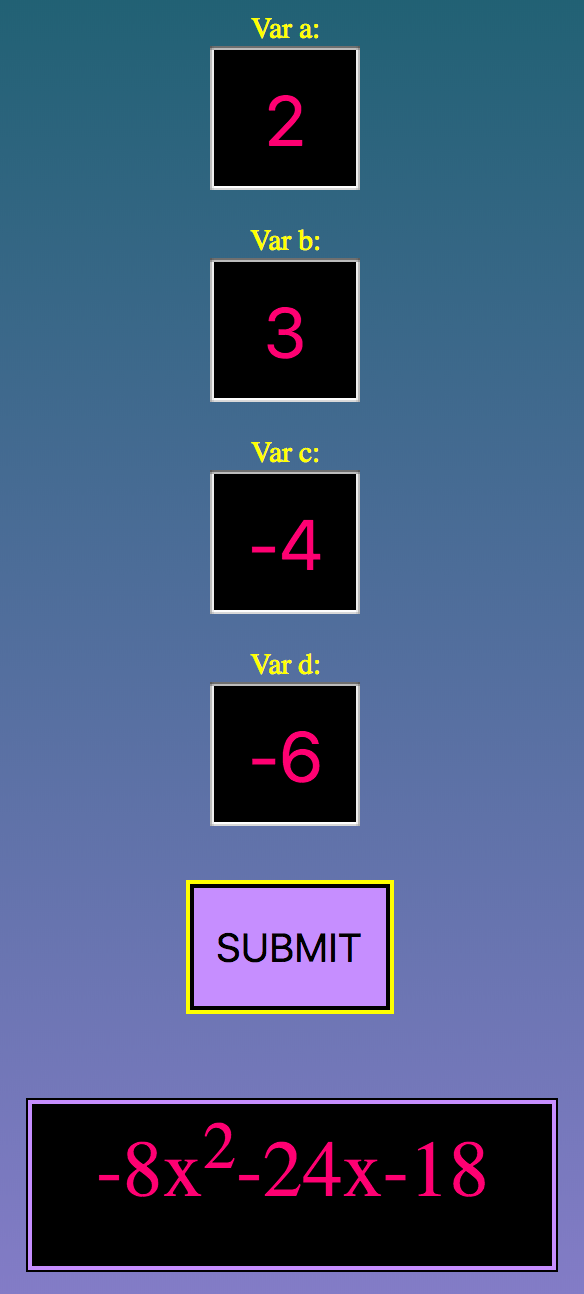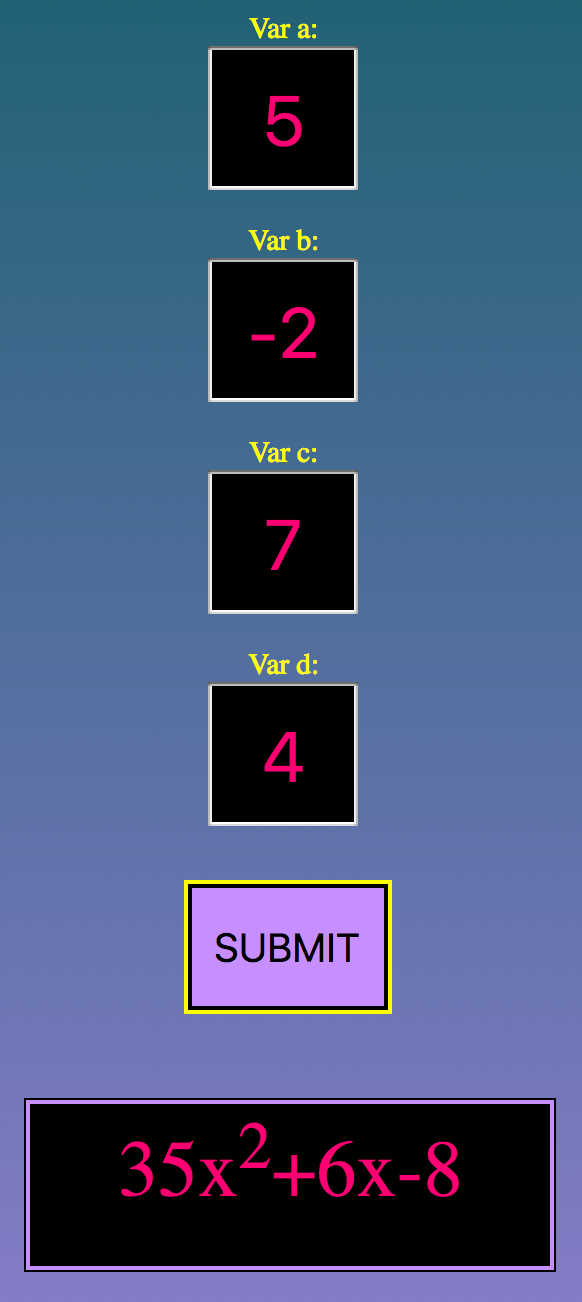LET'S DO FOIL!!

(ax + b)(cx + d)

Examples of the types of problems this program will solve are shown below:

(3x - 2)(-4x + 3)

(-2x + 5)(6x - 3)

This program uses FOIL to simplify the product of two binomials.

When looking at your FOIL problem, reading from left-to-right, you should see four numbers. Those numbers are defined using variables a, b, c, and d, respectively.

Variables a, b, c, and d are not allowed to be zero.
If there is no number infront of the variable, like (x + 6), then you would want to re-write it as (1x + 6). Then type in the number 1 for that variable's value.

Type each of your values into the boxes below.

Var a = Var b = Var c = Var d =

Example Problem:
FOIL: (2x + 3)(-4x - 6)
Example Problem:
FOIL: (5x - 2)(7x + 4)